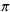Create a new printableGeometry
Math Worksheets

Sample - Click above to make a new math worksheet (PDF).
 Name _____________________________Date ___________________
Perimeter and Area
Find the area of each circle. State your answer in terms ofand also round your answer to the nearest tenth.

1.
 diameter = 30 cm

2.

3.
 diameter = 7.68 m

4.

5.
 78
mm

6.

7.
diameter =
 15
mm

8.
 diameter = 35.86 m

9.

10.
 diameter = 21.5 cm

11.

12.
 diameter = 42.68 m

13.

14.
diameter =
 87
mm

15.

16.
 diameter = 27.18 mm

17.
 diameter = 22 m

18.
 34
m

19.

20.
 diameter = 51.12 mm

21.

22.
 diameter = 35.24 m

23.
diameter =
 83
m

24.

25.

26.
 diameter = 40.84 m

 27 * This is a pre-made sheet.Use the link at the top of the page for a printable page.